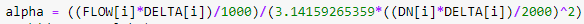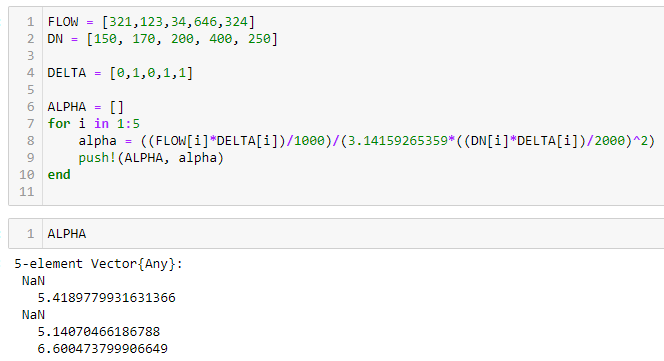# Problem in my object function because of NaN in my vector

Hi, all!

I have this code, already working great.
My objective function is
@NLobjective(model, Min, sum(total_price[i] for i in 1:21))

When I add a new constraint, this total_price vector assumes some NaN values and then the otimization return this:

It atributes NaN to all my variables because can’t sum the values with NaN.

There is a way to substitute these NaN, inside JuMP, for zero so the sum works?

Thank you a lot for your help!!

Do you know where the NaNs come from? Maybe we should avoid this situation altogether.

I have binary variables, and I use them to calculate each price.
Total_price is the sum of price+price+price.
When the variable is 0, the price[i] returns NaN

I tried to use isnan(x), but it requires the use of conditions and the JuMP doesn’t recognize them.
I was searching for some function/way that substitute NaN for zero without using if’s.

Why does price become NaN?

This is almost certainly a modeling error that you should fix. Checking for isnan wont work because it is non differentiable. Can you provide a reproducible example?

2 Likes

Ow, okay. I see.

I was trying to do an example smaller and I identify that error.
The error is that I use some equations like that:DELTA is my variable. When DELTA==0, the denominator become zero.

Trying to write an example closer to my code, it would be something like

using DataFrames, XLSX, JuMP, Ipopt, Juniper
optimizer = Juniper.Optimizer
nl_solver = optimizer_with_attributes(Ipopt.Optimizer, “print_level” => 0)
model = Model(optimizer_with_attributes(optimizer, “nl_solver”=>nl_solver))
@variable(model, DELTA[1:5], Bin)
FLOW = [321,123,34,646,324]
DN = [150, 170, 200, 400, 250]
ALPHA =
for i in 1:5
alpha = @NLexpression(model,((FLOW[i]DELTA[i])/1000)/(3.14159265359((DN[i]*DELTA[i])/2000)^2))
push!(ALPHA, alpha)
end
@NLconstraint(model, DELTA==0)
@NLconstraint(model, DELTA==0)
@NLobjective(model, Min, sum(ALPHA[i] for i in 1:5))
optim = optimize!(model)

If run just the base code, without the JuMP arguments, it appears the NaN.Do you know a way I can fix that? I cannot change the equations.

What we are saying is that you must change the equations. Your problem is not defined otherwise.

Why do you need `DELTA[i]` in the denominator? Isn’t it equivalent just to have `DELTA[i]` in the numerator only?

1 Like

I just created this example so you could understand the error. The equation in my code is way more complicated than that.
I’ll try to think a solution to that. Maybe the error is embedded to other point, not exactly that one.
Anyway, thank you a lot for your help!

1 Like

Can you provide the larger equation? Someone might have suggestions.

I think what has been said is that the solver cannot handle NaNs so we need to make sure that it never gets one. It doesn’t necessarily mean that we need to change the equations, but we may have to rewrite them. If the NaN is due to possible division by zero, you can introduce additional variables and rewrite division as multiplication, like num/den=t which means num=t*den. Basically this runs:

``````using JuMP, Ipopt, Juniper

FLOW = [321,123,34,646,324]
DN = [150, 170, 200, 400, 250]
ALPHA = []

optimizer = Juniper.Optimizer
nl_solver = optimizer_with_attributes(Ipopt.Optimizer, "print_level" => 0)
model = Model(optimizer_with_attributes(optimizer, "nl_solver"=>nl_solver))
@variable(model, DELTA[1:5], Bin)
####New here#################
@variable(model, aux[1:5]>=0) ######New variable
@NLexpression(model,num[i=1:5],((FLOW[i]*DELTA[i])/1000)) #####Numerator
@NLexpression(model,den[i=1:5],3.14159265359((DN[i]*DELTA[i])/2000)^2) #####Denominator
@NLconstraint(model,auxCstr[i=1:5],num[i]==aux[i]*den[i]) #####Multiplication instead of division - always feasible

for i in 1:5
push!(ALPHA, aux[i])
end
#############################
@NLconstraint(model, DELTA==0)
@NLconstraint(model, DELTA==0)

@NLobjective(model, Min, sum(ALPHA[i] for i in 1:5))
optim = optimize!(model)
``````

Other sources of NaN will have to be rewritten in a different way, though.

3 Likes